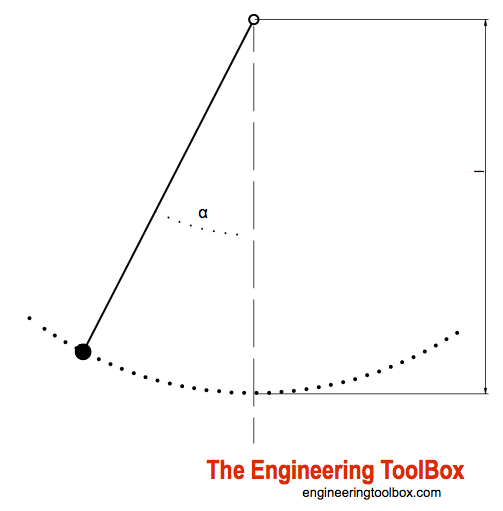Engineering ToolBox - Resources, Tools and Basic Information for Engineering and Design of Technical Applications!

# Pendulum

## A simple pendulum oscillates in the vertical plane due to gravity.

A simple - or mathematical - pendulum consists of a rigid body suspended from a fixed horizontal axis about which the body may oscillate in a vertical plane due to the action of gravity.The oscillating period for a simple pendulum can be calculated as

T = 2 π (l / ag)1/2              (1)

where

T = oscillating period for one cycle (s)

l = length of the pendulum (m, ft)

ag = acceleration of gravity (9.81 m/s2, 32.17 ft/s2)

### Example - Calculate the length of a pendulum with an oscillating period of 1 sec, 10 sec and 100 sec

The length of a pendulum with a given oscillating period can be calculated by transforming (1) to:

l = (T / (2 π))2 ag

Length for 1 sec - typically for an old fashion pendulum clock:

l = ((1 s) / (2 π))2 (9.81 m/s2)

= 0.249 (m)

Pendulum clocks were the world standard for accurate timekeeping for 270 years until the quartz clock was invented in 1927.

Length for 10 sec:

l = ((10 s) / (2 π))2 (9.81 m/s2)

= 24.9 (m)

Length for 100 sec:

l = ((100 s) / (2 π))2 (9.81 m/s2)

= 2487 (m)

### Four Types Of Pendulums

It is common to differentiate between four types of pendulums:

1. Compound or Physical Pendulum - A rigid body suspended from a fixed horizontal axis. The body may oscillate in a vertical plane due to the action of gravity.
2. Simple or Mathematical Pendulum - Similar to the compound or physical pendulum but the mass is concentrated in a single point and oscillates back and forth in the vertical plane. The mass point is connected to a horizontal axis with a weightless chord.
3. Conical Pendulum - Similar to the simple pendulum except that the weight suspended by the chord moves in uniform speed around the circumference of a circle in the horizontal plane.
4. Torsional Pendulum - A disk fixed to a slender rod. The rod is fastened to a fixed frame. If the disk is twisted it will oscillate back and forth about the axis of the rod.

## Related Topics

• ### Dynamics

Motion - velocity and acceleration, forces and torque.

## Related Documents

• ### Harmonic Oscillator

A simple harmonic oscillator.

## Engineering ToolBox - SketchUp Extension - Online 3D modeling!

Add standard and customized parametric components - like flange beams, lumbers, piping, stairs and more - to your Sketchup model with the Engineering ToolBox - SketchUp Extension - enabled for use with older versions of the amazing SketchUp Make and the newer "up to date" SketchUp Pro . Add the Engineering ToolBox extension to your SketchUp Make/Pro from the Extension Warehouse !

We don't collect information from our users. More about

## Citation

• The Engineering ToolBox (2012). Pendulum. [online] Available at: https://www.engineeringtoolbox.com/pendulum-d_1836.html [Accessed Day Month Year].

Modify the access date according your visit.

10.2.10